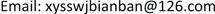1荥阳市水利局，河南 荥阳

2信阳师范学院，河南 信阳

3荥阳市税务局，河南 荥阳1. 引言

2. 圆周运动物体系统的向心力与离心力是作用力与反作用力的依据

3. 匀速圆周运动物体系统在受约束离心力作用下产生周期性运动3.1. 构建物理实验模型

3.2. 匀速圆周运动系统离心力作用的解析3.2.1. 匀速圆周运动系统离心力及在x轴方向上的冲量

F R = − F ′ R ,

F x = F R sin ϕ = − F ′ R sin ϕ = − m v 2 R sin ϕ .

∵ v x = v cos ϕ ， v x 1 = v cos ϕ 1 ， v x 2 = v cos ϕ 2 ， t = R ϕ v ；

∴ ∫ t 1 t 2 F x d t = F ¯ x ( t 2 − t 1 ) = ∫ t 1 t 2 ( − m v 2 R ) sin ϕ d t = ∫ ϕ 1 ϕ 2 ( − m v 2 R ) sin ϕ d ( R ϕ v )                           = m v cos ϕ 2 − m v cos ϕ 1 = M v 2 ( M ) − M v 1 (M)

F ¯ x = m v cos ϕ 2 − m v cos ϕ 1 t 2 − t 1 .

∫ 0 T 2 F x d t = F ¯ x T 2 = F ¯ x π R v = m v cos ϕ 2 − m v cos ϕ 1 | 0 π = − 2 m v

F ¯ x = m v cos ϕ 2 − m v cos ϕ 1 t 2 − t 1 = − 2 m v 2 π R .

v 2 ( M ) = m v cos ϕ 2 − m v cos ϕ 1 k m = − 2 m v k m = − 2 k v .

∫ T 2 T F x d t = F ¯ x T 2 = F ¯ x π R v = m v cos ϕ 2 − m v cos ϕ 1 | π 2 π = 2 m v

F ¯ x = m v cos ϕ 3 − m v cos ϕ 2 t 3 − t 2 = 2 m v 2 π R .

3.2.2. 离心力在x轴上的分量所做的功

∫ x 1 x 2 F x d x = F ¯ x ( x 2 − x 1 ) = ∫ x 1 x 2 ( − m v 2 R ) sin ϕ d x = ∫ x 1 x 2 ( − m v 2 R ) sin ϕ d ( R sin ϕ ) = 1 2 m v 2 cos 2 ϕ 2 − 1 2 m v 2 cos 2 ϕ 1 = 1 2 M v 2 ( M ) 2 − 1 2 M v 1 ( M ) 2

W = ∫ a b | f ( x ) d x | = ± ∫ a b f ( x ) d x

W | 0 π = ∫ 0 π 2 | F ′ x d x | + ∫ π 2 π | F ′ x d x | = 1 2 m v 2 + 1 2 m v 2 = 1 2 M v M 2 − 1 2 M v 0 ( M ) 2

∫ x 1 x 2 F x d x = F ¯ x [ x 2 ( M ) − x 1 ( M ) ] = F ¯ x X M = m v 2 = 1 2 M v 2 ( M ) 2 = 1 2 k m ( − 2 v k ) 2 = 2 k m v 2 ，

X M = 1 2 M v 2 ( M ) 2 F ¯ x = 2 k m v 2 π R 2 m v 2 = π R k ，

X M = π R 2 .

3.2.3. 后半周(π→2π)区间离心力在x轴上的分量做的功和振幅

| W | | π 2 π = ∫ π 3 π 2 | F ′ x d x | + ∫ 3 π 2 2 π | F ′ x d x | = 1 2 m v 2 + 1 2 m v 2 = − [ 1 2 M v M 3 2 − 1 2 M v M 2 ] .

F ¯ x = m v cos ϕ 3 − m v cos ϕ 2 t 3 − t 2 = − 2 m v 2 π R .

。(同上半周)

3.2.4. 前后半周(0→2π)做功之和

W = W | 0 π − | W | | π 2 π = 0 .

4. 轴对称 [<xref ref-type="bibr" rid="hanspub.33103-ref6">6</xref>] 圆弧弯道水流离心力对河道张力作用的解析算法

M = N m = n l m ，

∫ − l l F x n d l = n ∫ ϕ 1 ϕ 2 F ′ R cos ϕ d ( R ϕ ) = n ∫ ϕ 1 ϕ 2 m v 2 R cos ϕ d ( R ϕ ) = M l v 2 ( sin ϕ 2 − sin ϕ 1 ) = 2 M l v 2 sin ϕ 2 .

2012年7月24日长江三峡发生7万m3洪峰流量的罕见洪水，夹杂着泥沙直扑三峡大坝。如流经圆弧河槽段，平均河宽1000 m，平均河深5 m，圆弧河段有效长1000 m，有效半径5000 m， 2 ϕ = 17 ∘ 27' ，平均流速14 m/s，该段圆弧弯道水流沿x轴方向对河槽的张力：

∫ − l l F x n d l = n ∫ ϕ 1 ϕ 2 F ′ R cos ϕ d ( R ϕ ) = 2 M l v 2 sin ϕ 2 = 195673.5   N = 19 .57 ( 吨 ) 。

5. 结论